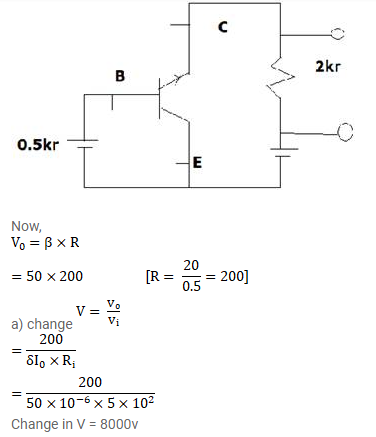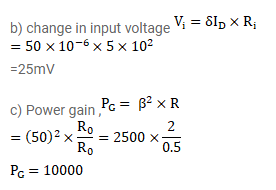# A load resistor of 2kΩ connected in the collector branch of an amplifier circuit

Question:

A load resistor of $2 \mathrm{k} \Omega$ connected in the collector branch of an amplifier circuit using a transistor in common-emitter mode. The current $\mathrm{B}=50$.the input resistance of the transistor is $0.50 \mathrm{k}$. If the input current is changed by $50 \mu \mathrm{A}$. (a) By what amount does the output voltage change, (b) by what amount does the input voltage change and (c) what is the power gain?

Solution: